Bates College Calculus Placement Exam

1) What is dy/dx if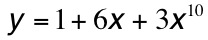? (2 Points)

a)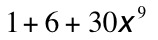b)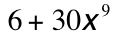c)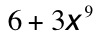d)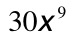2) What is dy/dx if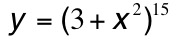? (2 Points)

a)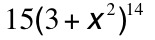b)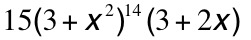c)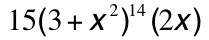d)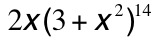3) What is dy/dx if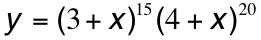? (2 Points)

a)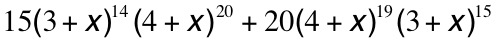b)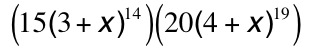c)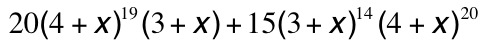d)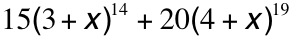4) What is dy/dx if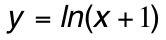? (2 Points)

a)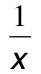b)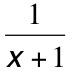c)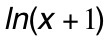d)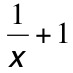5) What is dy/dx if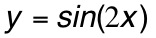? (2 Points)

a)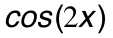b)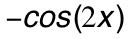c)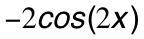d)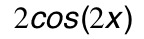Questions 6 through 11 refer to the graph shown. It is a graph of a bug's velocity (not position) as it crawls along a straight wire. When velocity is positive, the bug is moving to the right; when velocity is negative, the bug is moving to the left.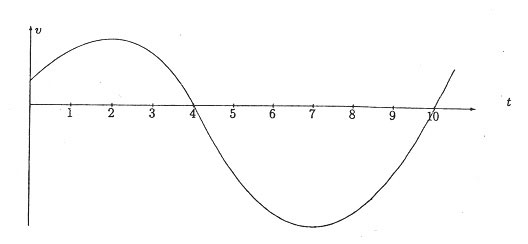6) At which time (or times) is the bug's velocity zero? (1 Point)

a) t=2

b) t=4

c) t=2 and t=7

d) t=4 and t=10

7) At which time or times does the bug change direction? (1 Point)

a) t=2

b) t=4

c) t=2 and t=7

d) t=4 and t=10

8) At which time is the bug moving most rapidly to the right? (1 Point)

a) t=1

b) t=2

c) t=7

d) t=9

9) How does the bug's position at time t=10 compare to its position at t=0? (1 Point)

a) At t=10, the bug is in the same place as at t=0.

b) At t=10, the bug is to the right of where it was at t=0.

c) At t=10, the bug is to the left of where it was at t=0.

d) We do not have enough information to answer this question.

10) At which time is the bug farthest to the right? (1 Point)

a) t=2

b) t=4

c) t=7

d) t=10

11) At which time or times is the bug's acceleration zero? (1 Point)

a) t=2

b) t=4

c) t=2 and t=7

d) t=4 and t=10

12) Which of the following is an antiderivative of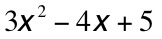? (2 Points)

a)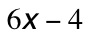b)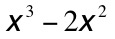c)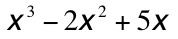d)13) For what value of x in the interval [0,2] is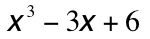the smallest? (2 Points)

a) x=0

b) x=0.5

c) x=1

d) x=2